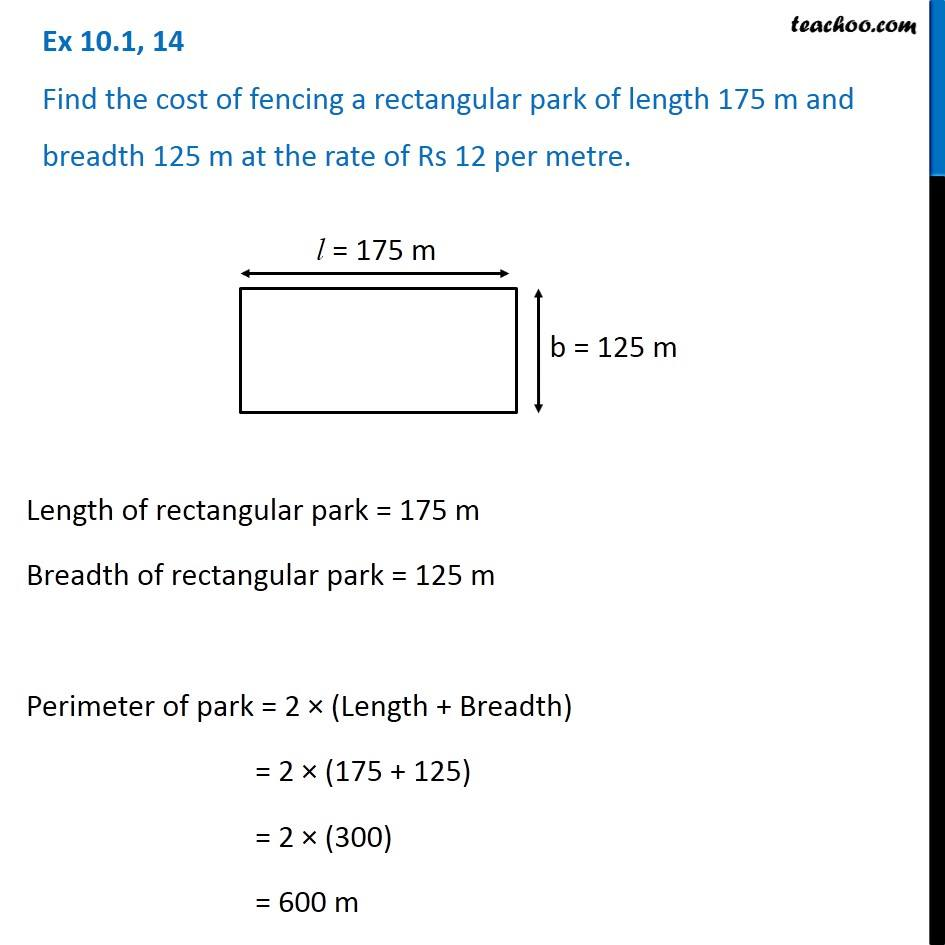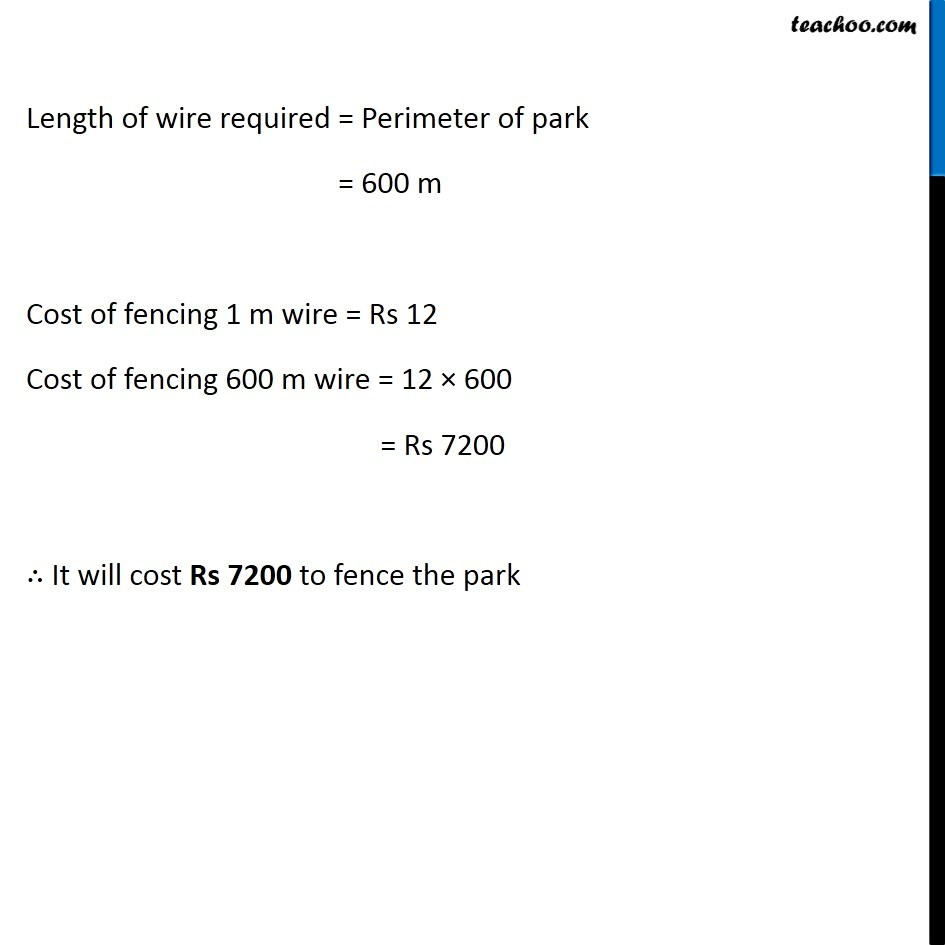Subscribe to our Youtube Channel - https://you.tube/teachoo

1. Chapter 10 Class 6 Mensuration
2. Concept wise
3. Perimeter of Rectangle

Transcript

Ex 10.1, 14 Find the cost of fencing a rectangular park of length 175 m and breadth 125 m at the rate of Rs 12 per metre. Length of rectangular park = 175 m Breadth of rectangular park = 125 m Perimeter of park = 2 × (Length + Breadth) = 2 × (175 + 125) = 2 × (300) = 600 m Length of wire required = Perimeter of park = 600 m Cost of fencing 1 m wire = Rs 12 Cost of fencing 600 m wire = 12 × 600 = Rs 7200 ∴ It will cost Rs 7200 to fence the park

Perimeter of Rectangle